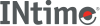﻿ nextafterINtime SDK Help
nextafter

Computes the next representable floating-point value following `x` in the direction of `y`. Thus, if `y` is less than `x`, nextafter shall return the largest representable floating-point number less than `x`. The nextafter function shall return `y` if `x` equals `y`.

```#include <math.h>

double nextafter (double x, double y);
```

#### Parameters

`x`, `y`
Double-precision floating-point values.

#### Return Values

• Upon successful completion, these functions shall return the next representable floating-point value following `x` in the direction of `y`.
• If `x`== `y`, `y` (of the type `x`) shall be returned.
• If `x` is finite and the correct function value would overflow, a range error shall occur and +/-HUGE_VAL (with the same sign as `x`) shall be returned.
• If `x` or `y` is NaN, a NaN shall be returned.
• If `x`!= `y` and the correct function value is subnormal, zero, or underflows, a range error shall occur, and either the correct function value (if representable) or 0.0 shall be returned.

#### Requirements

Versions Defined in Include Link to
INtime 3.0 intime/rt/include/math.h math.h clib.lib